﻿ Finite Element Modeling of Regular Waves by 1-D Madsen and Sorensen Extended Boussineq Equations

### Finite Element Modeling of Regular Waves by 1-D Madsen and Sorensen Extended Boussineq EquationsOPEN ACCESSPEER-REVIEWED

## Finite Element Modeling of Regular Waves by 1-D Madsen and Sorensen Extended Boussineq Equations

Parviz Ghadimi1,, Amin Rahimzadeh1, Mohammad H. Jabbari1, Rahim Zamanian2

1Department of Marine Technology, Amirkabir University of Technology, Tehran, Iran

2International Campus – Mechanical Engineering Group, Amirkabir University of Technology, Tehran, Iran

### Abstract

In this paper, finite element modeling of one-dimensional extended Boussinesq equations derived by Madsen and Sorensen is presented for simulation of propagating regular waves. In order to spatially discretize the finite element equations, method of weighted residual Galerkin approach is used. Discretization of third-order derivative in momentum equation is performed by introducing of an auxiliary equation, which makes it possible to use linear finite element method. Adams-Bashforth-Moulton predictor-corrector method is used for time integration. Regular wave trains are simulated using the proposed numerical scheme. For validation of the developed code, the model is applied to several examples of wave propagation over the computational domain and the obtained results of the current computations are compared against the experimental measurements. In all cases, the proposed model has proved very suitable for simulating the propagation of wave indicating favorable agreements with experimental data.

### At a glance: Figures

1234
Prev Next

• Ghadimi, Parviz, et al. "Finite Element Modeling of Regular Waves by 1-D Madsen and Sorensen Extended Boussineq Equations." American Journal of Marine Science 1.1 (2013): 1-6.
• Ghadimi, P. , Rahimzadeh, A. , Jabbari, M. H. , & Zamanian, R. (2013). Finite Element Modeling of Regular Waves by 1-D Madsen and Sorensen Extended Boussineq Equations. American Journal of Marine Science, 1(1), 1-6.
• Ghadimi, Parviz, Amin Rahimzadeh, Mohammad H. Jabbari, and Rahim Zamanian. "Finite Element Modeling of Regular Waves by 1-D Madsen and Sorensen Extended Boussineq Equations." American Journal of Marine Science 1, no. 1 (2013): 1-6.

 Import into BibTeX Import into EndNote Import into RefMan Import into RefWorks

### 1. Introduction

By propagating waves from the deep sea up into the surf zone, free surface water wave can be transformed because of presence of various barriers in its path and also due to the effect of different phenomena such as reflection, refraction, diffraction and shoaling. An accurate estimation of wave behavior in these situations can be very helpful to the marine structure designers not only to present a cost-effective design but also to provide human safety that are the most important aspects of the design process. The study of wave hydrodynamics dates about a century back when the first theories for linear and nonlinear wave motions developed more in the interest of the science than design and applications in the oceans. Among them, the Stokes theory for nonlinear water waves can be mentioned. Proposing a realistic model can be achieved in various ways such as observation of field data, laboratory setting with scale models in wave tanks or mathematical and numerical methods. Before the advent of high speed computers, experimental and analytical studies were the only tools in this field. In recent years, however; numerical methods have been widely used for accurate simulating of water waves propagating toward near shore.

Numerical models and computer simulations are the only tools available to understand in detail and predict the evolution of complex environmental systems. While the incompressible Navier-Stokes equations would provide an accurate mathematical model of these processes, the numerical solution of a realistic problem would involve a large three-dimensional spatial domain with a free-surface boundary. For both simplicity and reduction in time of computing, numerical models based on Boussinesq-type equations can be considered. Boussinesq-type models with depth averaged equations and reduced dimension in computational domain, a three-dimensional physical model in two-dimensional space and a two-dimensional physical model in one-dimensional space can be solved which, compared with the Navier-Stokes models, are much more economical.

There have been many attempts to solve the Boussinesq type equations by different methods of discretization. Finite difference method is one of the primal techniques which is employed to solve these equations. The first application of the finite difference method to the Eulerian form of the equations was done by Peregrine . In order to overcome difficulties associate with implementing finite difference method, finite element schemes were applied, mainly due to its geometrical flexibility. Several FEM methods have been introduced for the standard Boussinesq equations. Peregrin’s  standard Boussinesq equations were solved by Antunes Do Carmo et al. , Amborsi and Quartapelle , and again by Sherwin and Eskilsson [16, 17]. Beji and Nadaoka’s  derived extended Boussinesq equations, was solved by Li et al . The improved Boussinesq equations of Madsen and Sorensen  were also solved by Eskilsson and Sherwin [16, 17], Walkley and Berzins [19, 20], and Sorensen et al . For solving Boussinesq-type equations, finite volume techniques have also been implemented by several authors. Some works include those performed by Bradford and Sanders , Stansby , Erduran et al.  and Ning et al . It is clear that most of these applications have been considered within the last decade.

The goal of the current study is to model the improved system of Boussinesq equations derived by Madsen and Sorensen. Discretization of the equations is performed using non-overlapping elements in the context of the Galerkin finite element method with linear interpolating functions. A new scheme is applied to discretize the third order term in momentum equation. For implementation of the linear Galerkin finite element method, third order term is eliminated by introducing an auxiliary equation. Method of derivation of auxiliary equation in this work is different from that offered by Sorensen et al . This will be described in detail in Section 3. Discretization of time integrations in the governing equations is performed using predictor-corrector scheme proposed by Adams-Bashforth-Moulton. Finally, in order to validate the proposed scheme, the model is applied to some example tests of numerical wave propagating at different boundary conditions. Results of the developed code and the experimental measurements are presented for regular wave trains.

### 2. Derivation of Equations

Following equations present enhanced Boussinesq equations derived by Madsen and Sorensen. The first equation is related to continuity and the second equation to momentum equations.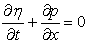(1)(2)

The z coordinate varies between the free surface and the sea bed h(x) with the origin taken at the still-water depth. The bottom boundary is assumed to be fixed and impermeable. Here η is the local free surface elevation measured from the still water level. d is the total water depth determined by h + η and P is the depth-integrated velocity component. B is a dispersion coefficient. Following Madsen and Sorensen, B is set to 1/15 which best represents the shallow water linear dispersion.

### 3. Numerical Model

3.1. Galerkin Finite Element Method

As pointed out earlier, discretization of spatial derivatives is performed using Galerkin finite element method. Solving the system with a finite element method requires that the horizontal domain be partitioned by elements to approximate the solution. For a typical finite element inside the domain Ω, all the unknowns are assumed to vary as(3)

where fi is the vector of nodal unknowns and Ni is the shape function corresponding to the ith nodal degree of freedom fi of element e.

3.1.1. Solution Formulation

Application of the enhanced Boussinesq equations derived by Madsen and Sorensen and implementation of the Galerkin finite element is presented in this section. Utilization of an auxiliary equation is discussed in detail. Introducing this auxiliary equation is due to the presence of the third order derivative in momentum equation, which makes it possibile to implement linear Galerkin method. Accordingly, momentum equation is rewritten by introducing w, defined by equation, as an auxiliary variable producing the system.(4)(5)(6)

where hx is the derivative of the water depth with respect to spatial coordinate x. In order to acquire stable solution, simple linear elements and linear interpolation implemented are used for spatially discretizing the variables p, η and w. Equation (6) is an auxiliary equation which is achieved based on the assumption that the nodal values are constant over all finite elements.

It should be mentioned that the method of obtaining an auxiliary equation for elimination of the third order derivative in momentum equation, is different from that proposed for two dimensional Madsen and Sorensen extended Boussinesq equations by Sorensen et al . To achieve this goal, they have rewritten the dispersive terms using the mild-slop assumption and modified these terms using linear long wave approximations. Subsequently, by spatial differentiation and combination of these equations, an auxiliary equation is obtained.

Proposed technique in this paper is similar to work by Li et al  based on the two dimensional improved Boussinesq equations derived by Beji and Nadaoka , without any essential for above assumptions and re-extracting the equations to acquire an auxiliary equation. As it can be understood, proposed technique here is easily applicable and also efficient and can be extended easily into two-dimensional domain.

3.1.2. Spatial Discretization

By applying Galerkin method, the continuity equation becomes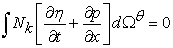(7)

By discretizing every term of Eq.7, the matrix equation for an element can be obtained as in(8)

where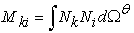(9)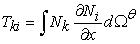(10)

Similar integral equations can also be written for Eqs.5 and 6 as in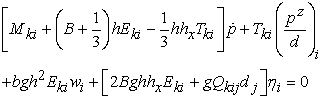(11)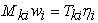(12)

where(13)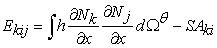(14)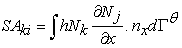(15)

Here, Γθ denotes the boundary of the element with unit normal n. These boundary integrals are needed only when natural boundary conditions are specified on particular part of the computational domain boundaries. If Dirichlet boundary conditions are specified at the boundaries, the boundary integrals are eliminated. The integrals (9), (10) and (13) through (15) are evaluated over each element individually and results are summed up at the nodes.

3.2. Time Integration

Here, we discretize our systems with respect to time, to integrate numerically in time from one time step to another. There are several time integration methods that have been proposed. In this work, in order to discretize time derivatives in equations method of Adams-Bashforth-Moulton predictor-corrector is considered. Matrix form of the assembled global equations corresponding to Eqs. 8 and 11, are as follows(16)

Here M is the coefficient matrix, f = η or p and E is a known vector which is calculated from the known values of η, p and w. By implementing the Adams-Bashforth predictor step, initial value of dependent variables can be achieved at the moment.(17)

where Δt is the adopted time step, n (n = 1, 2, . . . ,N 1,N) is number of time steps, and the known values of the right hand side are obtained from calculations of previous time steps. To obtain a high degree of convergence within each time step, the overall accuracy of time discretization procedure is increased by implementing Adams-Moulton corrector step, whereis calculated from the predictor step.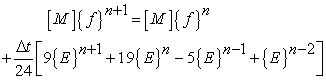(18)
3.3. Boundary Condition

To close the system of equations given in the former sections, we should seek to impose accurate boundary conditions at physical boundaries. Here, application of two different types of boundary conditions is considered; incidents wave boundary and a wave absorbing boundary. Detailed discussion on boundary conditions is presented in the following sections

3.3.1. Wave Absorbing Boundary

Suitable damping boundary condition is required for the numerical experiments. Accordingly, sponge layers are employed to eliminate the boundary reflections positioned next to the lateral boundaries at outflow. Damping process after each time step is performed by dividing fluxes and free surface elevation by a coefficient μ(x) which is defined as follows: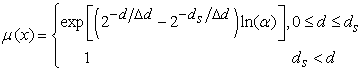(19)

Here, d is distance between the point on the sponge layer and the boundary, Δd is the typical dimension of the elements, damping layer width; ds, is usually equal to or twice of the wave lengths, and α is a constant to be determined which is considered α = 4 in this work.

3.3.2. Incident Wave Boundary

In order to numerically model the propagation of incident wave, it is necessary to apply an inflow boundary condition. Free surface profile of the first node at inflow boundary can be obtained at each time step as follows: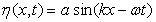(20)

Incident wave velocity can be determined by implementing linear theory [ 26] as in(21)

where h(x) is the still water depth from the seabed, α is the wave amplitude, ω is angular frequency and k is the wave number. Here, initial free surface profile is set to be zero all over the domain.

### 4. Test Cases

4.1. Propagation of Regular Wave Over Constant Depth

In order to show the capability of the improved Boussinesq equations derived by Madsen and Sorensen, the proposed technique along with the introduced auxiliary equation is utilized to simulate the propagation of a regular wave over a constant depth. The efficiency of damping procedure using sponge layer at the right site of the domain is investigated. To this end, the computation domain of length L=15m was divided into 250 elements with the finite element mesh size of Δx = 0.06m. Regular wave with initial amplitude of a = 0.025m and wave period of 0.85s is propagated over the computational domain. A parameter of kh = 3.14 is used in this test which happens to be in the range of applicability of the extended Boussinesq equation used in the current study. Water depth is considered h = 0.56m, which corresponds to a wave number k = 5.61. The results for free surface elevation is presented in Figure 1.

Figure 1 shows the progression of free surface profile at t=40s for regular wave along the length of the domain. It is seen that a steady periodic flow has been established and efficient damping process of outgoing wave has been achieved by implementing the sponge layer at the lateral boundary of outflow. As evidenced in this figure, proposed numerical technique is capable of simulating regular wave over a constant depth.

4.2. Propagation of Regular Wave Over Submerged Bar

In this section, the geometric flexibility with propagation of regular wave over submerged bar in using the proposed method is demonstrated. In order to assess the ability of the model for accurately simulating the nonlinear and dispersive wave effects in the shallow water, the input wave is propagated over a submerged bar. This problem has been studied previously by Luth et al . The bathymetry is presented in Figure 2.

The input wave on an undisturbed depth of h = 0.4 m is generated. The computation domain with length of L=30m was divided into 600 elements with the finite element mesh size of Δx = 0.05m. Inside the domain two slopes are applied at the left and right hand of the bar which have steepness of 1:20, 1:10, with lengths of 4.0m and 3.0m, respectively. The first slope starts at x = 6.0 m. Length of the crest of the bar is 2.0m. Incident wave with a wave period of T = 2.02 s, a wave amplitude of a = 0.01 with a wave number of k = 1.68 is generated. Sponge layer with length of 3.0m is positioned next to the right boundary. The periodic wave is propagated with time increment of Δt = 0.025s until the time t = 36 s. The comparison of the computed results against the experimental data for gauge stations at x = 2, 4, 10.5, 12.5, 13.5, 14.5, 15.7 and 17.3 m are illustrated in Figures 3 through 10 which display favorable agreements.

Figure 10. Free surface time history at x = 17.30 m: — numerical method; . . . experimental data

As wave propagates toward the bar, free surface elevation maintains its shape without any change until it reaches the bar. At the left side of the bar, the wave changes its shape in steep mode due to nonlinear shoaling. At the crest of the bar bound harmonics release as free harmonics decompose the wave into shorter waves, which results in a rapidly varying wave profile behind the bar. For correct propagation, the shorter wave components require a highly accurate dispersion relation. Therefore, this experiment has been used as a benchmark test for dispersive wave models.

As shown in Figs.3 through 10, an excellent agreement has been achieved against the available data, with minimal differences in surface profile at all gauge locations. Numerically computed time series of free surface profile at almost all gauge positions show no time lag, compared with the experimental time histories. Apart from the gauge stations at x = 13.5 and 14.50, which under-predict the time histories of numerical results, the proposed technique is shown to match the experimental time histories very well at the other gauge stations, compared against the experimental data. Finally, taking into account the fact that the enhanced Boussinesq equations are known to be weakly nonlinear and dispersive, numerical results achieved by the proposed technique can be considered quite suitable.

### 5. Conclusion

Finite element modeling of regular waves using an improved Boussinesq equations derived by Madsen and Sorensen is presented. The proposed numerical technique uses an auxiliary equation which makes it possible to use linear finite element method. One of the most significant findings to emerge from this study is the fact that it proposes a new procedure equivalent to lowering the order of third order derivative in momentum equation by introducing an auxiliary equation. It is shown that the proposed technique is easily applicable and efficient and accordingly can be extended to two dimensional models. Considering the proposed method, extended Boussinesq of Madsen and Sorensen equations are discretized in space using linear Galerkin finite method. Discretizing time integrations is performed using predictor-corrector method proposed by Adams-Bashforth-Moulton. In the first step, dependent variables at the moment predicted and accuracy of the discretizing process is increased in corrector step. Two types of boundary conditions such as incidents wave boundary and a wave absorbing boundary is applied. To validate the developed code, a propagating regular wave over a constant depth and a propagating regular wave over a submerged bar are simulated. The obtained results in the form of free surface time histories are compared with available experimental measurements and it has been demonstrated that the proposed model is capable of offering favorable agreements. Two dimensional modeling of Madsen and Sorensen Boussinesq equations using proposed technique is currently being extended and will be the subject of future paper.

### References

  Peregrine D. H., “Long waves on a beach,” J. Fluid Mech, 27. 815-827. 1967.In article CrossRef  Witting, J.M., “A unified model for the evolution of nonlinear water waves,” J. Comput. Phys, 56. 203-236. 1984.In article CrossRef  Madsen P. A., Sch¨affer H. A., Higher-order Boussinesq type equations for surface gravity waves: Derivation and analysis, Proc. R. Soc. London, 3123-318, 1998.In article  Madsen P. A., Sørensen O. R., “A new form of the Boussinesq equations with improved linear dispersion characteristics: Part 2. A slowly-varying bathymetry,” Coastal Eng, 18. 183-204. 1992.In article CrossRef  Nwogu O., “Alternative form of Boussinesq equations for nearshore wave propagation,” Coastal Eng, 15. 371-388. 1991.In article  Wei G., Kirby J. T., Grilli S. T., Subramanya R., “A fully nonlinear Boussinesq model for surface waves, Part 1: Highly nonlinear unsteady waves,” J. Fluid Mech, 294. 71-92. 1995.In article CrossRef  Lynett P. J., Liu P. L. F., “Linear analysis of the multi-layer model,” Coastal Eng, 35 51. 439-454. 2004.In article  Kennedy A. B., Kirby J. T., Chen Q., Dalrymple R. A., “Boussinesq-type equations with improved nonlinear behavior,” Wave Motion, 33. 225-243. 2001.In article CrossRef  Li B., “Equations for regular and irregular water wave propagation,” J. Waterway, Port,Coastal, and Ocean Eng, 134(2).121-142. 2008.In article  Gobbi M. F., Kirby J. T., Wei G., “A fully nonlinear Boussinesq model for surface waves, Part 2: Extension to O(kh)4,” J. Fluid Mech, 405. 181-210. 2000.In article CrossRef  Nadaoka K., Beji S., Nakagawa Y., A fully dispersive weakly nonlinear model for water waves, Proc. R. Soc. London, Ser. A, 453, 303-318, 1997.In article CrossRef  Madsen P. A., Murray R., Sørensen O. R., “A new form of the Boussinesq equations with improved linear dispersion characteristics,” Coastal Eng, 15. 371-388. 1991.In article CrossRef  Beji S., Nadaoka K., “A formal derivation and numerical modeling of the improved Boussinesq equations for varying depth,” Coastal Eng, 23(8). 691-704. 1996.In article  Antunes do Carmo J. S., Seabra Santos F. J., Barth´elemy E., “Surface waves propagation in shallow water: A finite element model,” Int. J. Numer. Meth. Fluids, 16. 447-459. 1993.In article CrossRef  Ambrosi D., Quartapelle L., “A Taylor–Galerkin method for simulating nonlinear dispersive water waves,” J. Comput. Phys, 146. 546-569. 1998.In article CrossRef  Eskilsson C., Sherwin S. J., “A discontinuous spectral element model for Boussinesq type equations,” J. Sci. Comput, 17. 143-152. 2002.In article CrossRef  Eskilsson C., Sherwin S. J., “Discontinuous Galerkin spectral/hp element modeling of dispersive shallow water systems,” J. Sci. Comput, 22. 269-288. 2005.In article CrossRef  Li Y. S., Liu S. X., Yu Y. X., Lai G. Z., “Numerical modeling of Boussinesq equations by finite element method, ” Coastal Eng, 37. 97-122. 1999.In article CrossRef  Walkley M., Berzins M., “A finite element method for the one-dimensional extended Boussinesq equations,” Int. J. Numer. Meth. Fluids, 29. 143-157. 1999.In article CrossRef  Walkley M., Berzins M., “A finite element method for the two-dimensional extended Boussinesq equations,” Int. J. Numer. Meth. Fluids, 39. 865-885. 2002.In article CrossRef  Ole R. Sørensen, Hemming A. Scha¨ffer, Lars S. Sørensen, “Boussinesq-type modelling using an unstructured finite element technique,” Coastal Eng, 50. 181-198. 2004.In article CrossRef  Bradford S. F., Sanders B. F., “Finite-volume models for unidirectional, nonlinear, dispersive waves,” J. Waterway, Port, Coastal, Ocean Eng, 128. 173-182. 2002.In article CrossRef  Stansby P. K., “Solitary wave run up and overtopping by a semi-implicit finite-volume shallow-water Boussinesq model,” J. Hydraulic Res, 41. 639-647. 2003.In article CrossRef  Erduran K. S., Ilic S., Kutija V., “Hybrid finite-volume 1 finite-difference scheme for the solution of Boussinesq equations,” Int. J. Numer. Meth. Fluids, 49. 1213- 1232, 2005.In article CrossRef  Ning D. Z., Zang J., Liang Q., Taylor P. H., Borthwick A. G. L., “Boussinesq cut-cell model for nonlinear wave interaction with coastal structures,” Int. J. Numer. Meth.Fluids, 57. 1459-1483. 2008.In article CrossRef  Dean R. G., Dalrymple R. A., Water Wave Mechanics for Engineers and Scientists, World Scientific, Singapore, 2000.In article  Luth H. R., Klopman G., Kitou N., Projects 13G: Kinematics of waves breaking partially on an offshore bar, LVD measurements for waves without a net onshore current, Technical Rep. No. H1573, Delft Hydraulics, Delft, the Netherlands. 1994In article